### 1，解方程组

• 2x + y = 3 —— ①
• 5x - 2y = 7 —— ②

• 4x + 2y = 6 —— ③

• 9x = 13 —— ④

### 2，线性回归模型

• y = 3 - 2x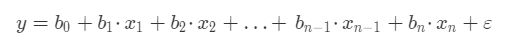• y 表示我们要预测的目标值。
• x1，x2…xn 代表每个特征，一共有n 个特征。
• b1，b2…bn 代表每个特征的系数，特征系数也代表了某个特征对目标值的影响。
• b0 是一个常数，称为截距。
• ε 表示模型的误差，也被称作损失函数。

1875 年，英国科学家弗朗西·斯高尔顿（达尔文的表弟）尝试寻找父代身高与子代身高之间的关系。

• Y = 0.516X + 33.73

### 3，线性拟合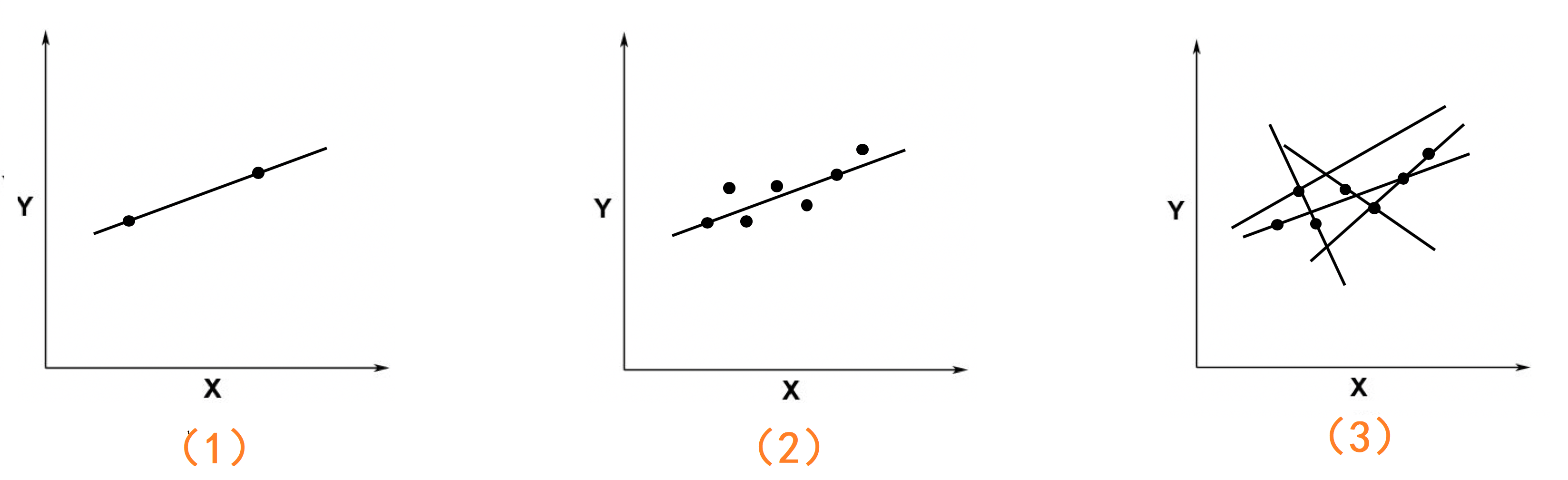• 在第1个图中只有两个点，这时候存在一条唯一的直线能够同时穿过这两个点，这条直线就是精确解。
• 当坐标中的点多于两个时，比如第2个图，这时候就不可能存在一条直线，同时穿过这些点。但是会存在多条直线，会尽可能多的穿过更多的点，就像图3。而这些直线中会有一条直线，是这些点的最好的拟合

### 4，最小二乘法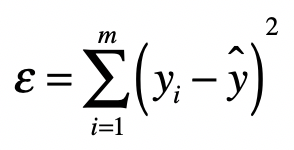• yi 是数据的真实值。
• y^ 是数据的预测值。
• ε 是我们要找的最小误差，它是所有的真实值与预测值之差的平方的和。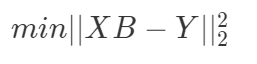• B 为系数矩阵
• X 为特征矩阵
• Y 为目标矩阵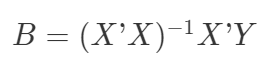• X'X 的转置矩阵。
• (X'X)-1(X'X) 的逆矩阵。

### 5，用 numpy 库进行矩阵运算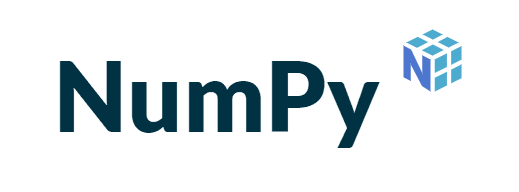NumPy 是一个使用Python 进行科学计算的软件包，其中就实现了我们需要的矩阵运算：

• x.transpose()：矩阵x 的转置运算。
• x.dot(y)：矩阵x 点乘矩阵y。
• x.I：返回可逆矩阵x 的逆矩阵。def get_B(X, Y):
_X = X.transpose()
B = (_X.dot(X)).I.dot(_X).dot(Y)
return B


0 1 1.4
1 -1 -0.48
2 8 13.2• b0​ + b1​⋅0 + b2​⋅1 = 1.4
• b0 ​+ b1​⋅1 - b2​⋅1 = −0.48
• b0 ​+ b1​⋅2 + b2​⋅8 = 13.2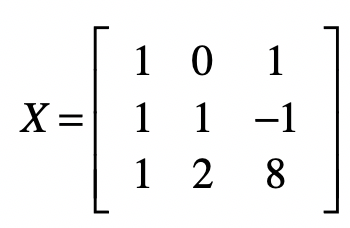from numpy import *

X = mat([
[1,0,1],
[1,1,-1],
[1,2,8]
])

Y = mat([[1.4],[-0.48],[13.2]])


>>> get_B(X, Y)
matrix([[-0.01454545],   # b0
[ 0.94909091],   # b1
[ 1.41454545]])  # b2


### 6，sklearn 对线性回归的实现

sklearn 库中的 LinearRegression 类是对线性回归的实现。

LinearRegression 类的原型：

LinearRegression(
fit_intercept=True,
normalize=False,
copy_X=True,
n_jobs=None)


• fit_intercept：拟合模型时，是否存在截距b0，默认为True，即存在。
• normalize：在拟合模型之前，是否要对特征集进行标准化处理。
• fit_interceptFalse 时，该参数被忽略。
• copy_X：是否复制特征集X
• n_jobs：用于计算的作业数，只对多重回归且比较复杂的数据进行加速。

0 1 1.4
1 -1 -0.48
2 8 13.2

X = [(0, 1), [1, -1], [2, 8]]
Y = [1.4, -0.48, 13.2]


from sklearn.linear_model import LinearRegression

reg = LinearRegression() # 均使用默认参数


reg.fit(X, Y)


coef_ 属性是特征系数列表：

>>> reg.coef_
array([0.94909091, 1.41454545])


intercept_ 属性是截距 b0 的值：

>>> reg.intercept_
-0.014545454545452863


• 决定系数 R2：该指标使用了回归平方和与总平方和之比，是反映模型拟合度的重要指标。
• 它的取值在 0 到 1 之间，越接近于 1 表示拟合的程度越好、数据分布越接近线性关系。
• 校正的决定系数 Rc2：如果特征非常多，那么Rc2 指标将更加可靠。

LinearRegression 类中的 score 方法就是R2 指标的实现：

>>> reg.score(X, Y)
1.0 # 结果是 1，说明特征集与目标集是非常好的线性关系。


### 7，对波士顿房价进行线性分析

from sklearn.datasets import load_boston

features = boston.data # 特征集
prices = boston.target # 目标集


from sklearn.linear_model import LinearRegression

# 在拟合之前对数据进行标准化处理
reg = LinearRegression(normalize=True)


reg.fit(features, prices)


>>> reg.score(features, prices)
0.7406426641094095


### 8，总结

（本节完。）